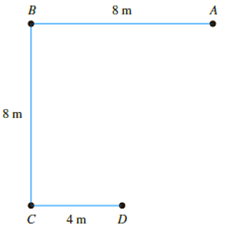Chapter 10, Problem 10.7PPrinciples of Geotechnical Enginee...

9th Edition
Braja M. Das + 1 other
ISBN: 9781305970939

Solutions

Chapter
SectionPrinciples of Geotechnical Enginee...

9th Edition
Braja M. Das + 1 other
ISBN: 9781305970939
Textbook Problem

Point loads of magnitude 125, 250, and 500 kN act at B, C, and D, respectively (Figure 10.40). Determine the increase in vertical stress at a depth of 10 m below the point A. Use Boussinesq’s equation.Figure 10.40

To determine

Calculate the increase in vertical stress at a depth of 10m below the point A by using Boussinesq’s equation.

Explanation

Given information:

The magnitude of load at B (PB) is 125kN.

The magnitude of load at C (PC) is 250kN.

The magnitude of load at D (PD) is 500kN.

The depth below point A (z) is 10m.

Calculation:

Calculate the distance (r) from A using the relation.

r=x2+y2 (1)

Here, x is the distance of a point from A along x axis and y is the distance of a point from A along y axis.

Show the location of points A, B, C, and D as in Figure 1.

Refer to Figure 1.

Calculate the distance (r) for point B from A as shown below.

Substitute 8m for x and 0 for y in Equation (1).

r=82+0=8m

Similarly, calculate the distance (r) of remaining points and tabulate as in Table 1.

Calculate the ratio rz as shown below.

For point B,

Substitute 8m for r and 10m for z.

rz=810=0.8

Similarly, calculate the ratio rz of remaining points and tabulate as in Table 1.

Calculate the value of influence factor (I1) as shown below.

Refer Table 10.1 “Variation of I1 for various values of rz” in the textbook.

Take the value of I1 as 0.1386 for the ratio rz of 0.8.

Similarly, take the value of I1 for the remaining points and tabulate as in Table 1.

Calculate the increase in vertical stress (Δσz) for any point using the relation.

Δσz=Pz2I1 (2)

Calculate the increase in vertical stress ((Δσz)B) for point B as shown below.

Substitute 125kN for P, 10m for z, and 0.1386 for I1 in Equation (2).

(Δσz)B=125102×0

Still sussing out bartleby?

Check out a sample textbook solution.

See a sample solution

The Solution to Your Study Problems

Bartleby provides explanations to thousands of textbook problems written by our experts, many with advanced degrees!

Get Started

Calculate the volume of material used in making 100 ft of 4 in. type K copper tubing.

Engineering Fundamentals: An Introduction to Engineering (MindTap Course List)

What do you think are the hallmarks of a good strategic plan?

Principles of Information Systems (MindTap Course List)

Determine the axial force in member BC of the frame. Note the joint D is a sliding pin.

International Edition---engineering Mechanics: Statics, 4th Edition

What types of injuries can occur to the ears during welding?

Welding: Principles and Applications (MindTap Course List)

Define the term, operating system. List popular operating systems for computers and mobile devices.

Enhanced Discovering Computers 2017 (Shelly Cashman Series) (MindTap Course List)